# What is FisherŌĆōYates shuffle in JavaScript?

Given an array, and generate the random permutation of array elements. It is similar like shuffling a deck of cards or randomize an array and every outcome after shuffling of array elements should likely and equally.

### Input output scenarios

Consider an array having some elements present in it and assume we have performed fisher yates shuffle on that array. The output array will be randomly shuffled and shuffles for every time we execute. All the permutations are equally and likely.

Input = [2, 4, 6, 8, 10]
Output = 4, 10, 6, 2, 8 // first iteration
8, 10, 2, 6, 4 // second iteration
2, 10, 8, 6, 4 // third iteration


Consider an arr[]. The easier way of solving is to create a temp[] array which is initially the copy of arr[]. Select an element randomly form temp[] and copy this element to arr and then delete the element in temp[]. Repeat the same process until every element is randomly selected from temp[] and moved to arr[].

### Algorithm

This algorithm performs swapping a random element in the array with the last element in the array over and again.

These are the steps to shuffle an array ŌłÆ

• Select a random index number in between the first and last index position in the given array.

• Now, swap the element with the last index element.

• Repeat the step one, but leave the element out of selection.

• Stop the shuffling when the first index is left in the random selection.

• Step one is repeated every time and last index of the previous random selection should be excluded from the selection.

HereŌĆÖs how fisher-yates array shuffle works ŌłÆ

To know how the is fisher-yates array shuffle working, letŌĆÖs assume an array arr=[10, 20, 30, 40, 50].

• From first index arr and last index position arr, select 30 at random and swap 30 and 50.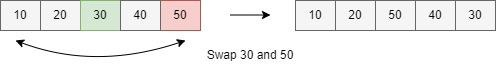• From first index arr and last index position arr excluding the previous selection.

• Select 20 at random and swap 20 and 40.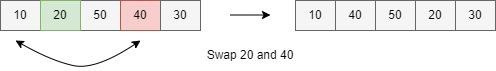• From first index arr to last index position arr excluding the previous selection.

• Select 30 at random, the swapping will not happen because the random selected index is not in between arr and arr.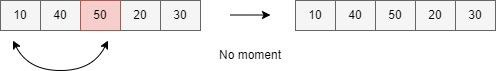• From first index arr to last index position arr excluding the previous selection.

• Select 10 at random, swap 10 and 40.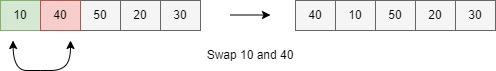• The final array after shuffling will be like in the below image.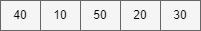### Example 1

In the example below, we have created an array with elements in it. Also created a while loop which will run as long as the array.length is greater than 0.

Array.length will be decremented by one each time whenever the while loop is repeated and also the last index is removed from the loop once it is swapped.

Elements will be swapped between temp and i. when the while loop iterates all the possibilities, it will print the output. The output will vary whenever you run the program again.

<!DOCTYPE html>
<html>
<title>Fisher-Yates shuffle in JavaScript</title>
<button onClick = "fisher_yates()"> Fisher Yates</button>
<p id = "para"></p>
<script>
function fisher_yates(){
let array = [40, 90, 50, 70, 80, 30, 60, 10, 20];
let i = array.length;
while (--i > 0) {
let temp = Math.floor(Math.random() * (i + 1));
[array[temp], array[i]] = [array[i], array[temp]];
}
document.getElementById("para").innerHTML = array;
};
</script>
<body>
</body>
</html>


### Example 2

In this below example, we have created an array having elements in it. Also created a for loop which will run as long as the array.length is greater than 0.

Array.length will be decremented by one each time whenever the for loop is repeated and also the last index is removed from the loop once it is swapped.

We have also created a button and the passed fy() function in it. Whenever the button is clicked, the loop starts running again and print shuffled array every time you click.

<!DOCTYPE html>
<html>
<body>
<button onclick="fy()">Try Fisher-Yates</button>
<p id="para"></p>
<script>
let Array = [25,1,5,10,40,100];
let len = Array.length;
let x;
function fy() {
for (x = len -1; x > 0; x--) {
var y = Math.floor(Math.random() * x)
var temp = Array[x]
Array[x] = Array[y]
Array[y] = temp
}
document.getElementById("para").innerHTML = Array;
};
</script>
</body>
</html>